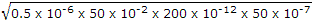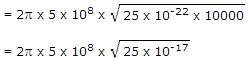# Electronics and Communication Engineering - Exam Questions Papers

46.
If the closed-loop transfer function T(s) of a unity negative feedback system is given by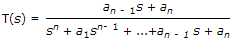then the steady state error for a unit ramp input is :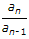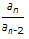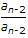Explanation: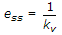.

47.
In 8085 microprocessor system, can an input and output port have same port address?
Yes
No
Insufficient data
None of these
Explanation:

Since microprocessor differentiates between an input or output operation on a port depending upon control signals RD and WR .

Therefore in a microprocessor system same port address can be given to two different input and output ports.

48.
5 skilled workers can build a wall in 20 days; 8 semi-skilled worker can build a wall in 25days; 10 unskilled workers can build a wall in 30 days, If a team has 2 killed, 6 semi-skilled and 5 unskilled workers, how long will it take to build the wall?
20 days
18 days
16 days
15 days
Explanation:

Given, 5 skilled workers can build a wall in 20 days i.e., 1 skilled worker can the wall build in 100 days

The capacity of each skilled worker is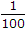8 semi-skilled workers can build a wall in 25 days i.e., 1 semi-skilled worker can build a wall in 200 days

The capacity of each semi-skilled worker is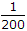Similarly, The capacity of 1 unskilled worker is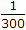Now, The capacity of 2 skilled + 6 semi-skilled + 5 unskilled workers is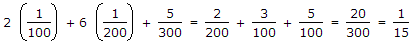The required numbers of days is 15.

49.
The fourier transform of the half cosine pulse as shown below is __________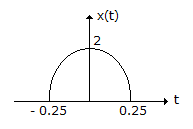0.5 {sin [0.5(f - 1)] + sin [0.5 (f + 1)]}
0.25 [sin c[0.25 (f - 2)1 + sin c[0.25(f + 2)]]
0.5 {sin c [0.5 (f - 1)] + sin c [0.5 (f + 1)]}
sin c(f - 0.5) + sin c (f + 0.5)
Explanation:

The given signal can be expressed as multiplication of x1(t) and x2(t) as shown below, where A = 2, T/2 = 0.25 ⇒ T = 0.5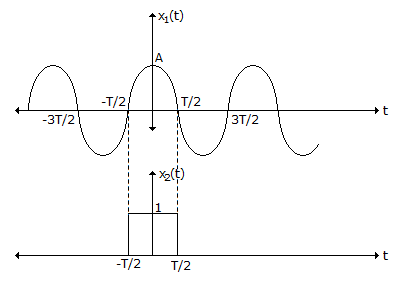x(t) = x1(t) x x2(t)

⇒ X(f) = X1(f) * X2(f)

Now X1(f) =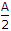[δ(f - f0) + δ(f + f0)]

where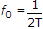and X2(f) = T . sin c[fT]

⇒ X(f) =[δ(f - f0) + δ(f + f0)]

*T. sinc(fT)

=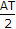[sinc[T(f - f0)] + sinc [T(f + f0)]]

Now, A = 2, T = 0.5

and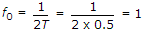⇒ X(f) = 0.5[sin c(0.5(f - 1)) + sinc(0.5(f + 1))].

50.
A lossless transmission line is 50 cm long and operates at a frequency of 500 MHz. Inductance and capacitances present in a line are 0.5 μH/m and 200 pF/m. Phase constant and phase velocity are respectively.
5p, 2p x 108 m/sec
5p, 108 m/sec
31.4, 108 m/sec
31.4, 2p x 108 m/sec
β = ωLC = 106 x 2p x 500 x